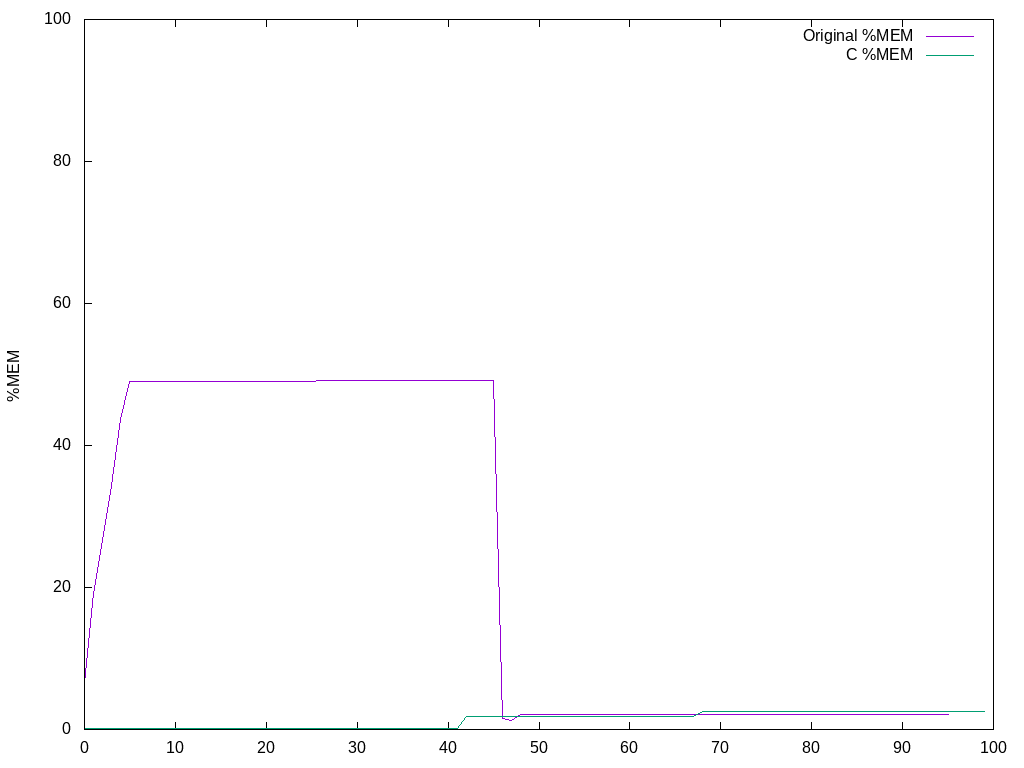I’ve been using my Glacier Push utility for a while now and it has been working well. But recently I noticed that on really large files the initial memory usage spiked, and then fell off. I suspected that the treehash calculation (implemented in Haskell) was not efficient so I re-implemented it in plain C.

The first thing was to write a utility to calculate the SHA256 of a buffer. Fortunately the OpenSSL docs have lots of examples to work from. Ignoring error handling, this is fairly straightforward:

The next thing is the tree hash algorithm. This is basically a Merkle tree on SHA256.

In short, we divide the file into 1Mb blocks and hash each of them. Then we hash pairs, pairs of pairs, and so on, until we have one block left. For example a 4Mb file would take two steps of hashing:We don’t need a full tree ADT because we have an upper bound on the number of blocks (the file on disk is fixed) so we can make do with a buffer for the hashes and a temp buffer for writing intermediate results. The algorithm boils down to this loop (full source is here):

Our C interface to treehash has this declaration:

To call this from Haskell we do some FFI:

And we can write a nice wrapper in Haskell by providing the file size:

To monitor the memory performance I logged the output of ps and plotted the results using gnuplot (scripts borrowed from Bruno Girin).

Memory usage (as percentage of 16Gb total memory) when pushing a 7.5Gb file to Glacier:The C version uses so little memory that it barely shows up on the plot. Both implementations use about the same amount of memory after the treehash calculation.